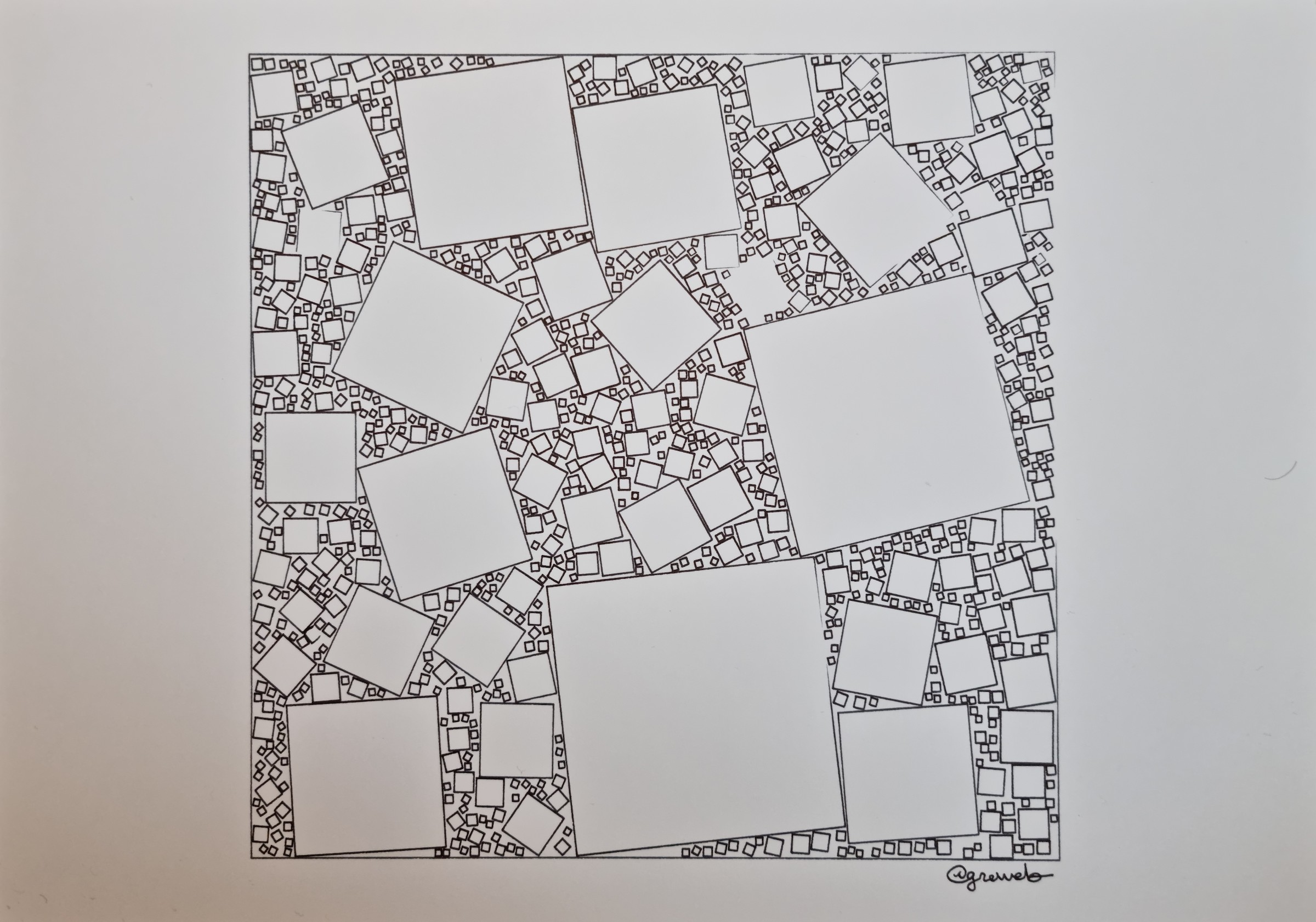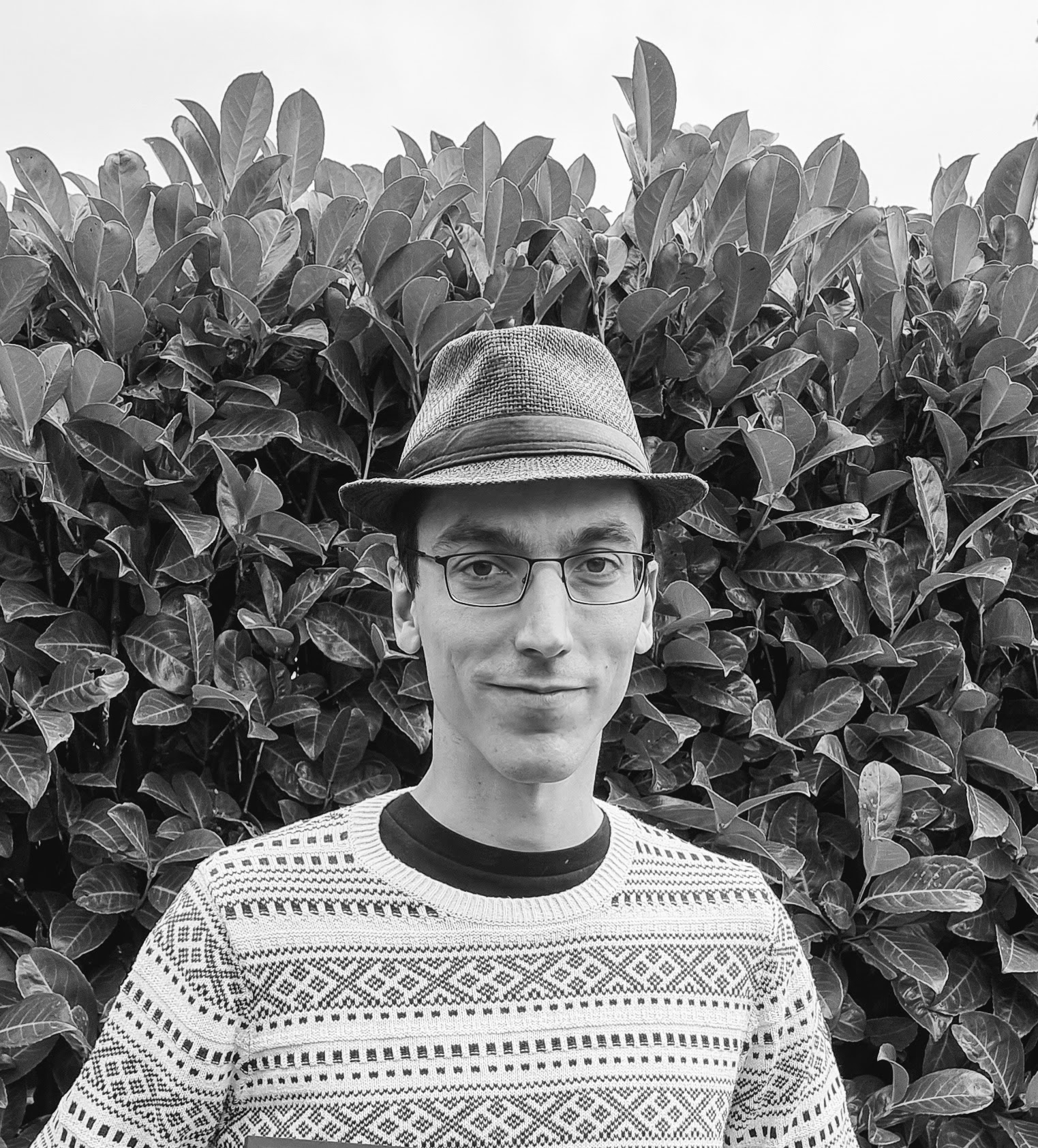# greweb.me / Plot #179 "squares packing 001"Packing rotated squares in a square. Black fountain pens on Bristol. Interestingly one square didn't draw due to ink issues. It makes the final art pretty unique!

## Technical notes

The algorithm brute-forces with 1 million iterations to find square location, for each iteration it will then search for the biggest square that can fit in the location space. I didn't even implemented dichotomic search for finding the square scale (it's a simple loop) but the overall script runs relatively fast in Rustlang (less than 10 seconds).

main loop

``````let mut polys = Vec::new();
let mut rng = rng_from_seed(opts.seed);
for i in 0..1000000 {
let x: f64 = rng.gen_range(bounds.0, bounds.2);
let y: f64 = rng.gen_range(bounds.1, bounds.3);
let a: f64 = rng.gen_range(0.0, 8.0);
if let Some(size) = poly_square_scaling_search(bounds, &polys, x, y, a, min_threshold) {
let poly = rotated_square_as_polygon(x, y, size - pad, a);
polys.push(poly);
}
if polys.len() > desired_count {
break;
}
}
``````

search

``````fn poly_square_scaling_search(
boundaries: (f64, f64, f64, f64),
polys: &Vec<Polygon<f64>>,
x: f64,
y: f64,
angle: f64,
min_threshold: f64
) -> Option<f64> {
let mut size = 0.1;
let dsize = 0.1;
// TODO dichotomic search could help perf here...
loop {
let poly = rotated_square_as_polygon(x, y, size, angle);
let bounds = poly.bounding_rect().unwrap();
let topleft: Point<f64> = bounds.min().into();
let bottomright: Point<f64> = topleft + point!(
x: bounds.width(),
y: bounds.height()
);
if out_of_boundaries(topleft.x_y(), boundaries) || out_of_boundaries(bottomright.x_y(), boundaries) {
break;
}
if poly_collides_in_polys(polys, &poly) {
break;
}
size += dsize;
}
if size < min_threshold {
return None;
}
return Some(size);
}
``````creative coder experimenting with GLSL shaders, Rust, and fountain pens robot plots. infinite noise explorer.